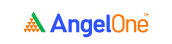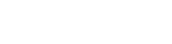# Deviation and distribution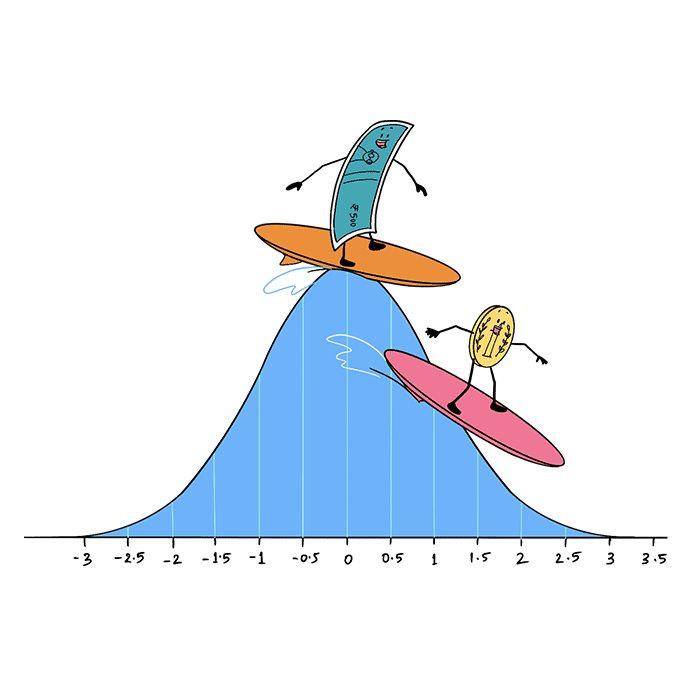Let’s connect the dots and make sense of what we saw in the earlier chapters. Here’s what we know so far.

There are three different variables that you can use to determine the correlation between two stocks:

• Differential
• Price ratio

And there are three basic statistical tools we can use to understand the distribution of a set of data points:

• Mean
• Median
• Mode

Why not put two and two together and calculate the mean, median and mode for the differential, spread and price ratio?

### Calculating the mean, median and mode for the three key variables

Up until now, we’ve been dealing with the closing prices of TCS and Infosys for a one-month period, for the sake of easier understanding. But to truly identify the correlation and the general behaviour of two stocks, it’s better to study their performance over a longer period, say 6 months or 1 year.

In fact, the longer the period, the more data points you have. And so, the more accurate your results and observations are likely to be.

So, here onward, let’s work with the 1-year data for the same two stocks. You can download this data from the NSE website. Taking the 1-year period from 15 Feb, 2020 to 15 Feb, 2021, we get 249 observations or data points. Using the formulas for mean, median and mode, you can calculate the metrics for the differential, the spread, and the price ratio for TCS and Infosys stocks over the 1-year period.

But of course, calculating these metrics for 249 data points isn’t just going to be hectic; it also provides plenty of room for human error. Anyway, why sweat it when you can just make use of a function in MS Excel and get the job done in a jiffy?

That’s just what we’ve done. Here, take a look at the screenshot showing you the mean, median and mode for the three key variables over the 1-year period.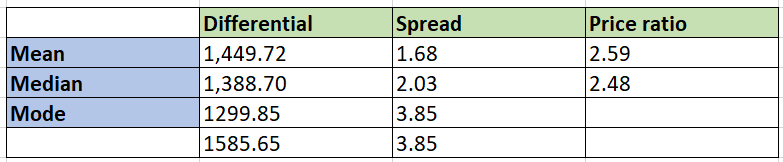mean

And just like how we did in the earlier chapter, we can also calculate the correlation between the following three variables for both these stocks over the 1-year period:

• The closing prices of the stocks
• The daily change in their closing prices
• The daily return they deliver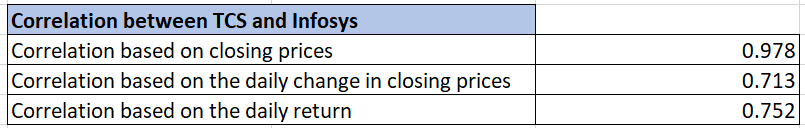Let’s look at a quick standard deviation example to understand. Say there’s a class of 30 students, and they all take a math test. The maximum marks in the test is 100.

Now, let’s say the average score of the 30 students comes out to be 53.4.

• The class topper, Ajay, scored 96
• Another student, Vijay, scored 62

Vijay’s score is closer to the class average of 53.4 than Ajay’s. In other words, Vijay’s score is more common, while Ajay’s is more uncommon. Also, this example clearly shows us that the data points in a set of observations tend to be spread out from the mean.

But what is the extent of that spread, or that deviation?

That’s what standard deviation helps us calculate. Statistically speaking, the standard deviation helps you measure the extent of deviation of a set of observations from their arithmetic mean.

You may have learned of the standard deviation formula in your high school. But now, since you’re using a spreadsheet to track all the data related to the pair of stocks, you may as well make use of the standard deviation calculator function in your spreadsheet.

For the data we’ve taken, here’s what the standard deviation calculator for the differential, spread and ratio shows.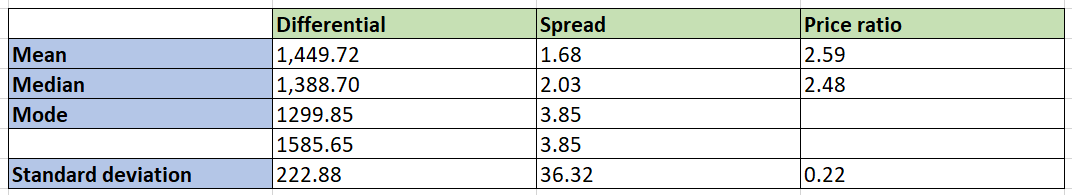So, how does the standard deviation play a role in pair trading? You see, given the volume of data and the resulting observations about the pair’s behavior, you can determine how much the latest price information for the present trading day deviates from what is normal for the stock. This helps you identify potential points to initiate a trade.

To get to that point, however, we’ll have to cover a few more concepts.

### Normal distribution and the bell curve

You’ve seen that the data points in a set of observations are distributed around the average. But what is the pattern in which the observations deviated from the average? More importantly, is there a pattern at all?

It turns out there are many patterns in which data can be distributed around the average. One such pattern is the normal distribution. According to this pattern, most of the data points tend to be concentrated around the area closer to the mean, with very few observations present towards the extremes.

Take the example we saw above. Vijay’s score (62) is more common, while Ajay’s (96) is more uncommon. After all, most students in your typical classroom tend to score closer to the average than scoring at either of the extremes - which is failing or getting a score that’s in the 90s.

The details of how data is spread out in the normal distribution can be explained by the empirical rule. You can make use of this to understand how to determine normal distribution.

### The empirical rule (aka the 68–95–99.7 rule)

According to this rule:

• 68% of values are within one standard deviation (1 SD) away from the mean.
• 95% of values are within two standard deviations (2 SD) away from the mean.
• 99.7% of values are within three standard deviations (3 SD) away from the mean.

Graphically, this is how the empirical rule is depicted.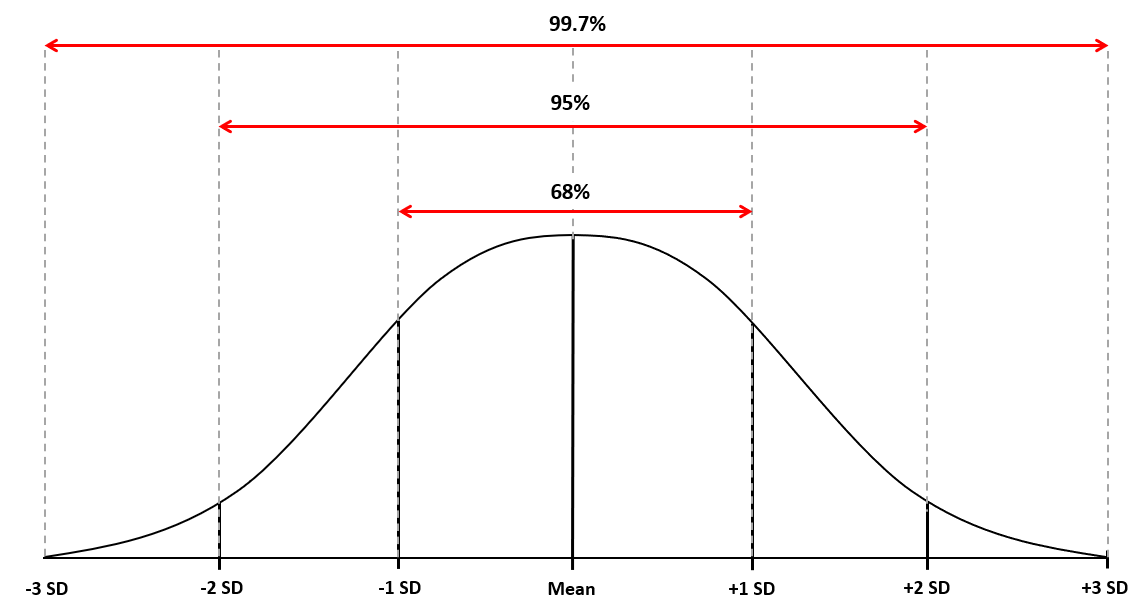These percentages also give this rule its alternate name, which is the 68-95-99.7 rule. And the curve you see here is popularly known as the bell curve.

Now, if you’re wondering what 1 SD, 2 SD and 3 SD are, here’s how we can arrive at these figures.

• +1 SD = mean + (1 x SD)
• +2 SD = mean + (2 x SD)
• +3 SD = mean + (3 x SD)
• -1 SD = mean - (1 x SD)
• -2 SD = mean - (2 x SD)
• -3 SD = mean - (3 x SD)

Using the empirical rule (or the normal distribution formula) for the differential, spread and ratio for the 1-year period discussed above, here’s what we get.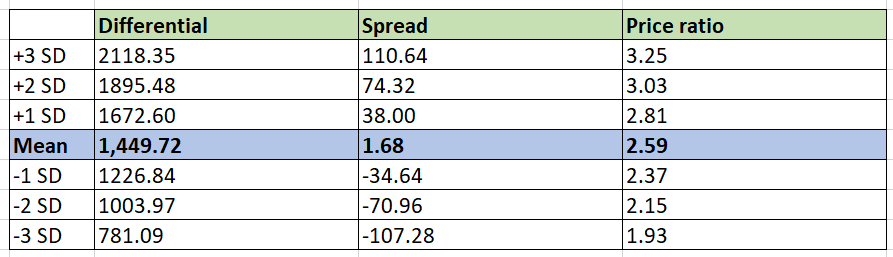### Gathering all the data calculated so far

So far, we’ve calculated the correlation between TCS and Infosys based on three different parameters, we’ve computed the mean, median and mode for the differential, spread and price ratio of these stocks, and we’ve applied the empirical rule to the three variables.

Let’s collate all that data in one space.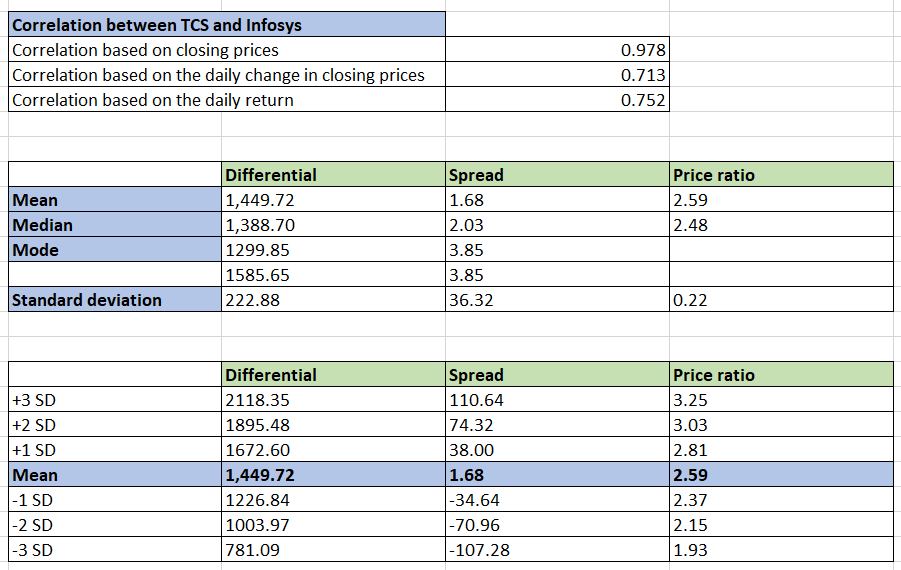#### Wrapping up

So, now that we have all the required inputs, we can move on to see what the density curve is, and how that can be used to identify when you can potentially initiate a trade.

#### A quick recap

• The standard deviation helps you measure the extent of deviation of a set of observations from their arithmetic mean.
• Given the volume of data and the resulting observations about the pair’s behavior, you can determine how much the latest price information for the present trading day deviates from what is normal for the stock. This helps you identify potential points to initiate a trade.
• According to the normal distribution pattern, most of the data points tend to be concentrated around the area closer to the mean, with very few observations present towards the extremes.
• The normal distribution is graphically represented by the bell curve.
• The curve shows you that 68% of values are within one standard deviation (1 SD) away  from the mean, 95% of values are within two standard deviations (2 SD) away from the mean and 99.7% of values are within three standard deviations (3 SD) away from the mean.Take the quiz for this chapter & mark it complete.

How would you rate this chapter?

Get Information Mindfulness!

Catch-up With Market

News in 60 Seconds.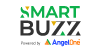The perfect starter to begin and stay tuned with your learning journey anytime and anywhere.